Open In App
Related Articles
• CBSE Class 11 Physics Notes

# Work Energy Theorem

The concept “work” is commonly used in ordinary speech, and we understand that it refers to the act of accomplishing something. For example, you are currently improving your understanding of Physics by reading this article! However, Physics may disagree on this point. The Work-energy Theorem explains why this Physics of no work exists!

When an operating force displaces a particle, work is said to be done. There is no work done if there is no relocation. You may become fatigued if you stand for an extended period of time, but according to Physics, you have done no labor.

### What is Work?

The work done on a system by a constant force is the product of the component of the force in the direction of motion times the distance through which the force acts.

Work done in one-dimensional motion can be expressed in equation form as

W = Fdcosθ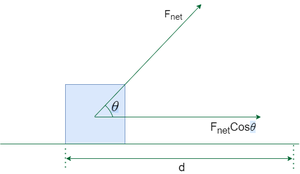where W is work, F is the magnitude of the force of the system, d the magnitude of the displacement of the system, and θ is the angle between the force vector F and the displacement vector d.

Work Done can be either Positive or Negative or even Zero.

• Positive Work: When a force moves an object in its direction, the work done is considered positive. Example: The motion of a ball falling towards the earth, where the displacement of the ball is measured, is an example of this type of work. The ball is moving in the direction of gravity.
• Negative Work: When force and displacement are in opposite directions, it is presumed that the work is negative. Example: When a ball is tossed upwards, for example, the displacement will be upwards; nevertheless, the force due to friction will be downwards. The earth’s gravity will be in a downward direction.
• Zero Work: The total work done by the force on the object is zero if the direction of the force and the displacement are perpendicular to each other. Example: When we push forcefully against a wall, for example, the force we are exerting on the wall is ineffective since the wall’s displacement equals d = 0.

### What is Energy?

The capacity to work is defined as energy. Some types of energy include potential energy, kinetic energy, thermal energy, electrical energy, chemical energy, and nuclear energy. Motion is linked to all sources of energy. When a body is in motion, for example, it has kinetic energy. When a tensed object, such as a bow or spring, is at rest, it has the capacity to create motion; its configuration holds potential energy. Nuclear energy is also potential energy because it arises from the arrangement of subatomic particles in an atom’s nucleus.

There are many forms of energy as mentioned above, Here we mainly focus on Kinetic energy.

Kinetic Energy:

• Kinetic energy is the type of energy that an object or particle has as a result of its movement.
• When work is done on an object by exerting a net force, the item accelerates and gains kinetic energy as a result.
• Kinetic energy is a property of a moving object or particle that is determined by its mass as well as its motion.
• If the motion along a path from one place to another, the kind of kinetic energy that the object possesses is Translational Kinetic Energy, if rotation about an axis, then the object possesses Rotational Kinetic Energy.
1. Translational Kinetic Energy:
• A body’s translational kinetic energy is equal to half of the product of its mass, m, and its velocity, v, or• This formula is only valid for particles moving at low to moderate speeds; for really high-speed particles, it produces values that are too small. When an object approaches the speed of light (3 × 108 meters per second, or 186,000 miles per second), its mass increases, requiring the application of the rules of relativity.
• The increase in mass of a particle over its rest mass multiplied by the square of the speed of light equals relativistic kinetic energy.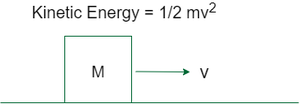A Body of Mass M and moving with Velocity V

• Rotational Kinetic Energy: The moment of inertia I, for a rotating body, relates to mass, while the angular velocity (omega), relates to linear, or translational velocity. As a result, rotational kinetic energy equals half of the product of moment of inertia and square of angular velocity or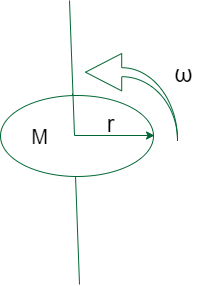A disc of Mass M and radius r rotating along its axis with Angular velocity ω

### Work-Energy Theorem

The statement of the Work energy theorem is,

Work-energy theorem states that the work done by the sum of all forces acting on a particle equals the change in the kinetic energy of the particle (or) The net work done on a system is equal to the change in Kinetic energy.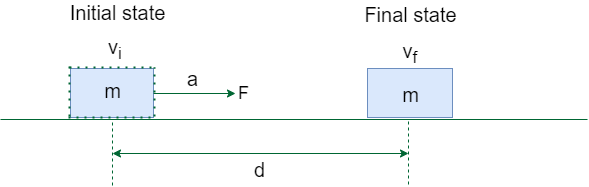Work-Energy Theorem

The work W done by the net force on a particle equals the change in the particle’s kinetic energy KE.where vi and vf are the speeds of the particle before and after the application of force, and m is the particle’s mass.

Derivation for Work Done by Constant Force

Let us will consider the case in which the resultant force F is constant in both magnitude and direction and is parallel to the velocity of the particle. The particle is moving with constant acceleration a, along a straight line.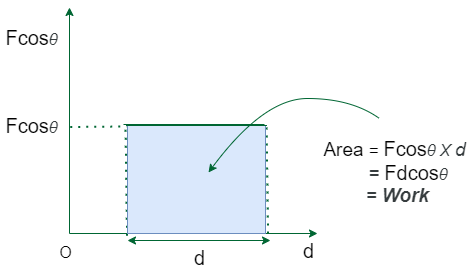Work done by a constant force

The relationship between the net force and the acceleration is given by the equation F = ma (Newton’s second law), and the particle’s displacement d, can be determined from the equation,(from the equationwhere v=final velocity, u=initial velocity and s = displacement)

We get,The work of the net force is calculated as the product of its magnitude and the particle’s displacement (W=Fd).

Substituting the above equations yields:W=Therefore, the net work done = change in Kinetic Energy

Derivation for Work Done by Variable Force

Variable forces are the most common forces we encounter on a daily basis. The work-energy formula for variable force is a bit complicated to figure out. Consider a graph with displacement on one axis and variable force on the other. The x-axis represents displacement, while the y-axis represents a force in this graph. Along the x-axis, divide the graph’s area into arbitrarily small width rectangles.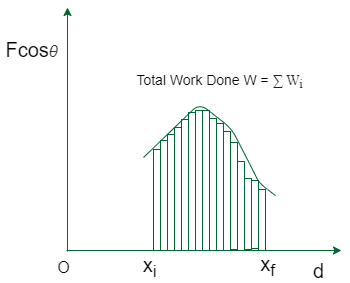Work done by a variable force

We can suppose that the force applied is constant for an arbitrarily tiny displacementx. As a result, we have;

⇒As a definite integral of force over the net displacement, we can now define work as:

⇒Because kinetic energy is equal to,

The change in kinetic energy over time can be described as follows:

⇒⇒Acceleration, according to Newton’s second law of motion, is the change in velocity of an object with respect to time. As a result, the above equation can be rewritten as,

⇒We can express the previous equation as, because F= ma and velocity is the rate of change of displacement over time.

⇒When both sides of the equation’s time derivatives are removed, we get

⇒ dK=Fdx

By integrating the previous equation along the x-axis, we get,

⇒⇒or we can write,Hence we get,The above equation is the proof of the work-energy theorem for the variable force.

### Sample Problem

Problem 1: A 2 kg ball is dropped from a height of 10 m. Calculate the work that has been done on the ball between the moment it is released and the moment when it hits the ground. Assume that air resistance can be neglected.

Solution:

Given,

Mass of the ball, m=2 kg.

Initial height of the ball, hi =10 m.

Final height of the ball, hf =0 m.

We must calculate the amount of work done on the ball as it hits the ground.

The ball is dropping freely here, conserving energy. We know that the difference in kinetic energy equals to the work done.

Because the ball is in a motionless condition when it is dropped, it has no kinetic energy at first.

All the ball’s potential energy is transformed to kinetic energy when the ball touches the ground.

So, Potential Energy of Ball at height hi is,

Ep = mghNow, The ball had 196 J of potential energy when it was released and 0 J of kinetic energy.

When the ball hit the ground, it had 0 J of potential energy and 196 J of kinetic energy.

Therefore, Initial Kinetic Energy KEi=0 J and KEf=196 J.

By work-energy theorem:

Wnet = ΔKE

KEf − KEi = 196−0

= 196 J

Therefore, The net work done on the ball is 196 J.

Problem 2: A car of mass 500 kg traveling at a speed of 16 m/s applies the car’s brakes at some point. The car’s brakes provide a frictional force of 4000 N. Determine the stopping distance of the car.

Solution:

Given,

Mass of the car, m = 500 kg

Speed of the car, v = 16 m/s

Frictional force of brakes,= 4000 N

Applying the work-energy theorem as:

The change in the kinetic energy is equal to the work done by the frictional force of the car’s brakes.

Therefore, Initial Kinetic energy of car at the moment brakes were applied is,⇒ 64 kJ.

As the car is at rest, Final Kinetic energy =0, therefore= 64 kJ.                                                                                     ……(1)

Consider the displacement of car is ΔX. Since the direction of the applied force and the displacement are in opposite directions, θ=180°.

Then the work done is given by:……(2)

By work-energy theorem, The change in kinetic energy is equal to the work done.Therefore, The stopping distance of car is 16 m.

Problem 3: On a rainy day, The driver of a car applied brakes seeing a red light. It is measured that the car stopped with 100 m skid mark behind it from the point of application of brakes, the coefficient of friction was estimated to be 0.45.  What was the speed of the car when the driver applied the brakes?

Solution:

Given,

Displacement of car, d = 100m

Coefficient of Friction, μ = 0.45

We know that, force of friction is the cause of the work done on car to slow it to a stop.  We also know that the work is equal to the change in kinetic energy.

−W=ΔKE                                (-ve symbol because Frictional force and the displacement are in opposite direction, cos(180°)=-1)Work is equal to the force times the displacement over which the force acted.

−W=Fd

Therefore,The force in this case is the force of friction. so, Ffriction = μFN

Since the car is on a level road the normal force is equal and opposite the force of gravity.

FN=FG

The force of gravity is equal to the mass times the acceleration due to gravity, FG=mg

Therefore, Ffriction = μmg

By, work-kinetic energy equation,Since the final velocity is 0 we get,Therefore, Initial velocity of car is 29.69 m/s.

Problem 4: The net propulsion force of a 2000 kg rocket is 600 N. The rocket accelerates uniformly from an initial velocity of 50 m/s to a final velocity of 80 m/s in a short amount of time. Assume that the rocket’s mass remains constant while it burns fuel and that the net force is directed in the direction of motion. How much net work has been done on the rocket (in KJ)?

Solution:

Given,

Mass of rocket, m = 2000 kg.

Net propulsion Force, F = 600 N.

Initial Velocity of rocket, vi = 50 m/s.

Final Velocity of rocket, vf = 80 m/s.

Applying Work Energy Theorem,

W = ΔKE

Initial Kinetic energy of the rocket KEi is given by:Similarly, Final Kinetic energy of rocket KEf is given by,Net Work done = The change in the kinetic energy at the two velocities:

W = ΔKE

6400000−2500000 =3 900000 J

Therefore, Net work done on the rocket is 3900 kJ.

Problem 5: A 100 g arrow is fired from a bow whose string exerts an average force of 150 N on the arrow over a distance of 50 cm. What is the speed of the arrow as it leaves the bow?

Solution:

Given,

Mass of arrow, m = 100g

Force exerted on the arrow, F = 150N

Displacement of string, d = 50cm

Applying Work-Energy Theorem,

W = ΔKEWork is equal to the force times the displacement over which the force acted.

W = Fd

Therefore,Substituting the values, we getTherefore, Velocity of arrow as it leaves the bow is 122.47 m/s.

Problem 6: Ronaldo kicked a football, and it traveled 50m with a constant velocity, how much work does Ronaldo do?

Solution:

Work is the product of a net force over a given distance.

W = Fnet d

There must be a net force exerted and a non-zero displacement of the object in order for it to do work.

The displacement is provided, but we must solve for the force.

According to Newton’s Second Law of Motion, the football moves at a constant velocity, which means its acceleration is zero.Since, vf=vi, a=0 m/s2

F = ma

= m(0)

= 0N

If the force is zero, then the work is also zero. Therefore, Work done, W is 0 J.

Problem 7: A 20 kg box is moving at 4 m/s. A net force of 100 N is constantly applied on the box in the direction of its movement until it has moved 25 m. What is the approximate final velocity of the box?

Solution:

Given,

Mass of box, m = 20kg

Initial Velocity of box, vi = 4 m/s

Force acting on the box, F = 100N.

Displacement of the box, d = 25m.

Applying Work-Energy Theorem,

W = ΔKEWork done by the block, W = Fd

W = 100 × 25 J

= 2500 J

Initial Kinetic Energy,Substituting the value, we get,Therefore, appropriate final velocity is 23.05 m/s.